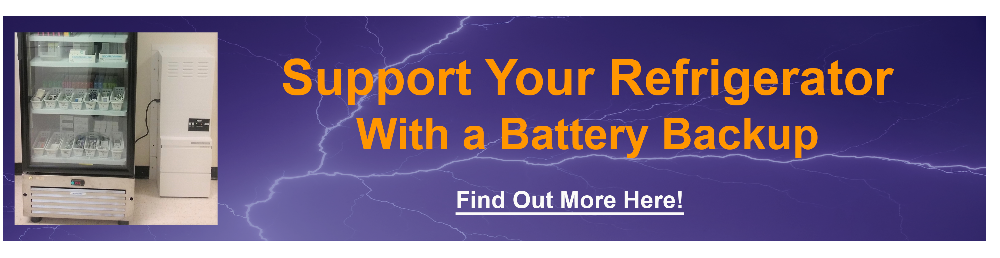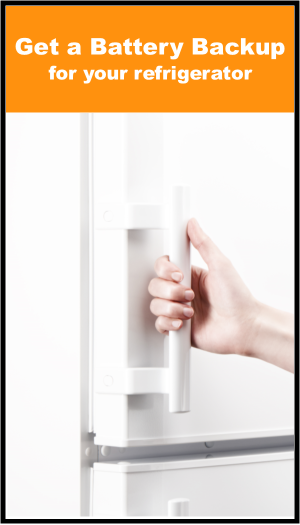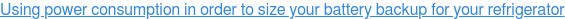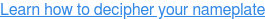CALL TOLL FREE1.800.765.3237

If you are trying to find out how many amps a refrigerator uses over time, the easiest

way to find out, is to google your refrigerators model number and see if it has an

Energy Star rating. If it has an energy star rating they usually list it out per year.

Example:  350KWH per year (that is 350,000 Watts hours per year)

# Using the Energy Star Rating to Figure Out the Average Power Consumption:

•    Using the example above:
•
•    If you take 350,000 and divide it by the number of days in a year (365) that will give you 958.91 watt hours per day:
•    ( 350,000 / 365 = 958.91 wh )
•
•   If you divide 958.91 watt hours by the number of hours in a day (24) you get 39.96 average running watts:
•   ( 958.91 / 24 = 39.96 )
•
•   If you divide the running watts by the voltage you will get the amps (Watts/Volts = Amps).  Most refrigerators in the US and Canada run    on 120 volts, so you take the 39.96 average watts and divide that by 120 volts you will find that the refrigerator averages .34 amps:
•   ( 39.96 / 120 = .34 )
•
•   *If your refrigerator does not have energy star rating (which most vaccine and commercial refrigerators do not) you will either have to      contact the manufacturer of the refrigerator (who may have this information) or purchase a KWH meter.  Having a KWH meter would        give you a more precise measurement anyway.### Buying an Energy Meter to Measure Power Consumption:An energy meter is a great easy way to measure your appliances power

consumption.  This works by plugging the meter into the wall outlet and then

plugging the refrigerator into the meter.  The meter will calculate the amount of

power the device is using over time.

These meters are generally inexpensive and may actually save you money on your

electric bill, by helping you become more aware of how much power your

appliances are using.

The picture link on the side of this page will direct where you can purchase one of

these.

The meter you purchase will likely come with user instructions, but they are

generally very easy to use.### Why is it Usually so Much Less Than the Nameplate Rated      Amperage?

The nameplate amperage is the amount of amps the appliance pulls when the

refrigerator’s compressor is running.  The compressor cycles on and off making the average way lower than the running

value.  The average will vary with the insulation value in the refrigerator.## What Are Refrigerator Amps?

Refrigerator amps are the amount of electrical current it’s compressor uses to cool it’s compartment. Amperage for most household refrigerators, is anywhere from 3 to 5 if the voltage is 120. A 15 to 20 amp dedicated circuit is required because the in-rush amperage is much higher. The average amperage is lower because the compressor isn’t running all the time, this is often measured in kilowatt hours KWH.

### If your Fridge Does Not Have an Energy Star. Try Doing a Calculation Using the              Nameplate Amperage:

Using a calculation based on the refrigerator, the nameplate amp rating is more or less an educated guess. Each

refrigerator has different efficiency levels.  Typically a refrigerator runs on 35% duty cycle, (65% for a freezer) meaning

that if you take that nameplate amperage and divide it by 35%, you can get an idea as to how much power the average

running amps will be.